# HDL Code Generation for Streaming Matrix Multiply System Object

This example shows how HDL Coder™ implements a streaming mode of matrix multiplication with configurable sizes.

### How to Multiply Matrices

Let A, B be two matrices then C= A * B is the matrix multiplication of A and B. If A is an m-by-p and B is an p-by-n matrix, then C is an m-by-n matrix defined by

```C(i,j) = A(i,1)B(1,j) + A(i,2)B(2,j) + -------- + A(i,p)B(p,j) ```

This inner definition says that C(i,j) is the inner product of ith row of A with the jth column of B

For non scalar A and B, the number of columns of A must equal to the number of rows of B

Example:

` A = [1 3 5;2 4 7]; (2 X 3 matrix)`
` B = [-5 8 11;3 9 21;4 0 8]; (3 X 3 matrix)`

After calculating inner product of rows of A with columns of B

` C = [24 35 114;30 52 162]; (2 X 3 matrix)`

### Introduction

Streaming Matrix Multiply supports multiplication of two matrices with configurable matrix sizes and dot product size. Dot product size is equal to the number of multipliers used for computation. This block can accepts serialized input data from matrix in row major or column major format.

### Matrix Multiply Interface: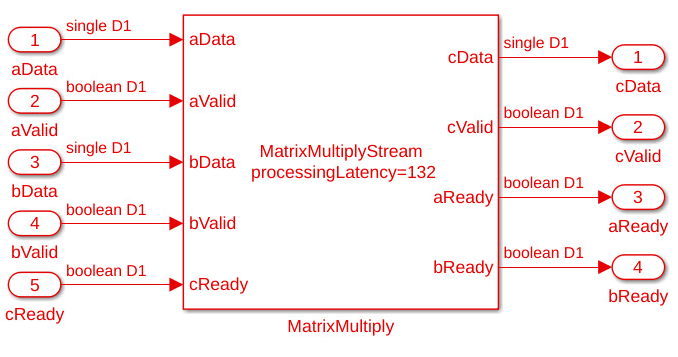Matrix Multiply ports description:### MatrixMultiply Implementation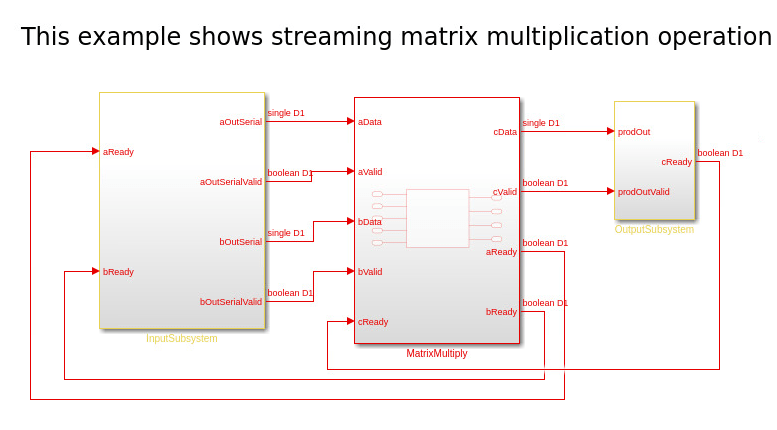This example model contains three subsystems: `InputSubsystem`, `MatrixMultiply` and `OutputSubsystem`. The `InputSubsystem` is the upstream module that serializes the matrix inputs(A,B) to the processing module when `aReady` and `bReady` signals are enabled. The `OutputSubsystem` is the downstream module that deserializes the data from the processing module to matrix output(C) when the `cReady` signal is enabled. The `MatrixMultiply` is a processing module that implements the matrix multiplication.

```open_system('hdlcoder_streaming_matrix_multiply_max_latency'); ```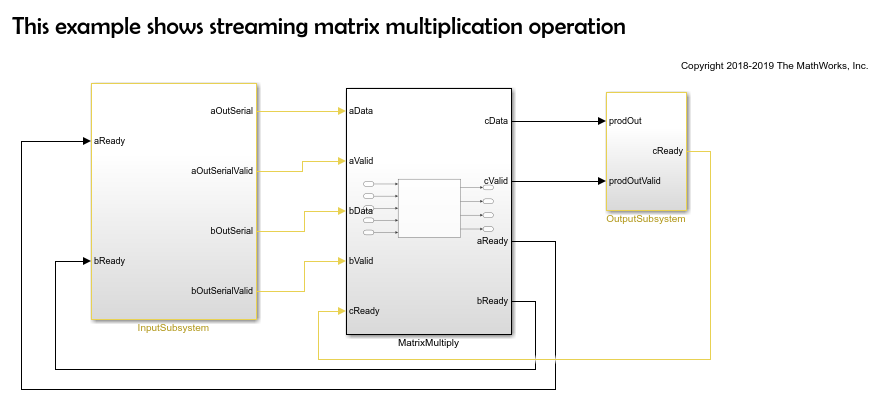### Matrix Multiply Timing diagrams

Timing diagramTiming diagramModelsim results for streaming matrix multiply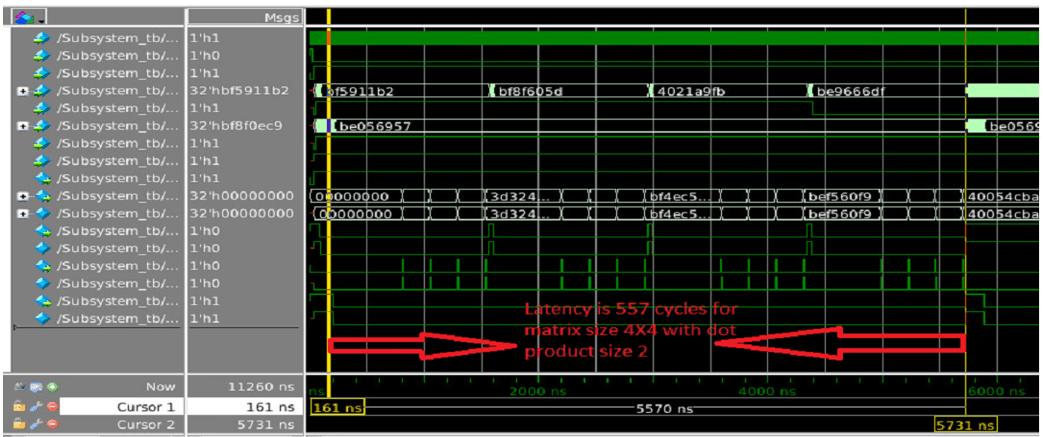### Matrix Multiply Block parameters: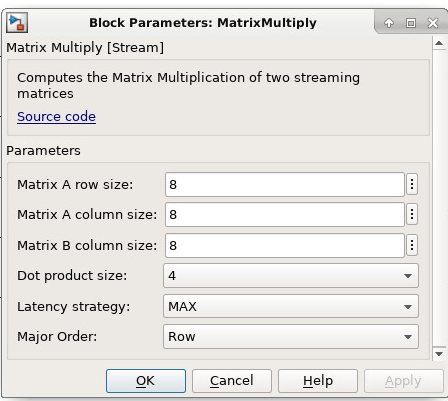```Matrix-A Row Size : Enter row size of input matrix A as a positive integer. Matrix-A Column Size : Enter column size of input matrix B as a positive integer which is equals to input matrix B row size. Matrix-B Column Size : Enter column size of input matrix B as a positive integer. Dot product size : Select dot product size from drop down menu(1,2,4,8,16,32,64) which should be less than input matrix A column size. LatencyStrategy : Select latency strategy from drop down menu ({'ZERO, 'MIN', 'MAX'}) which should be same as HDL coder latency strategy. Processing latency depends on the latency strategy. Major Order : Select row major or column major based on the input data streaming.```

### Matrix Multiply Block usage

1. Set block parameters of MATLAB System block.

2. Select input matrix sizes based on the values set in block parameters.

3. Generate HDL code for MatrixMultiply subsystem.

### Generated code and Generated model

After running code generation for MatrixMultiply subsystem, generated code will beGenerated model contains the MatrixMultiply MATLAB System block. During modelsim simulation code generation outputs are compared with MATLAB System block outputs.

### Synthesis statistics### Restrictions

• Matrix dot product sizes can be `1` or a power of `2`. The allowed sizes are `1`, `2`, `4`, `8`, `16`, `32` and `64`.

• Input data types of the matrices must be `single` and block must be used in the `Native Floating Point` mode.

• Input matrices must not be larger than 64-by-64 in size.

`mtimes`